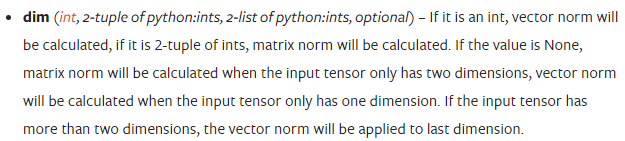# Torch.norm() fail to calculate 1-norm of matrix

When I calculate the default 2-norm of matrix on 2 dimensions, it works.However, when I calculate the 1-norm on these 2 dimensions, it fails.(Just add ‘p=1’)

The error is so evident, the dim here must be a int.
The 1-norm of matrix is the maximum-column sum of the matrix, see this.My input “a” and “b” are both 10x32x32 tensor, calculating the 1-norm of last two dimension, what I expect to get is a 10x1x1 tensor.

reference:
 Matlab Matrix 1-norm

result = torch.norm((a-b).view(10, -1), p=1, dim=1)

Well, thank you@Sunshine352, this is a good way to replace, and it seems enough for me. I will take it temporarily.
But it looks like different from the 1-norm definition? And the problem is still confuse me.